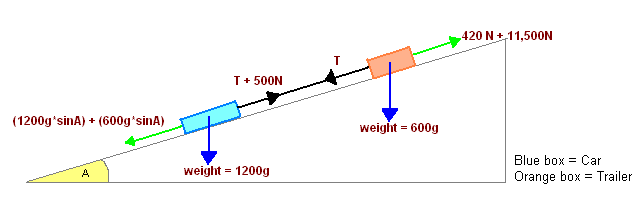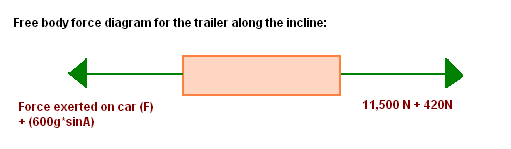A Level mechanics: Force exerted on a car by a trailer when brakes are applied

Hi :)

I have an A Level Mechanics 2 exam in 3 days. I managed to 'solve' this problem from my book, but I'm having some trouble with the underlying concepts. It would be really nice if someone could explain part (c) of the question to me (I've included my solution below but I don't understand how it works out). My book has next to no explanations and I'm self-studying for this exam, so I don't have access to any teachers either. Thank-you :)

Homework Statement

A car of total mass 1200kg is travelling along a straight horizontal road at 40ms^-1 when the driver suddenly applies the brakes. The brakes exert a constant force and the car comes to rest after travelling a distance of 80m. The other resistances on the car total 500N.

(a) Find the magnitude of the force exerted by the brakes on the car.

A trailer, with no brakes, is now attached to the car by means of a tow-bar. The mass of the trailer is 600kg, and when the trailer is moving, it experiences a constant resistance of magnitude 420N. The tow-bar is modelled as a light rod which remains parallel to the road at all times. The car and trailer come to a straight hill, which is inclined to the horizontal at an angle A, where sin A = 1/14. They move together down the hill. The driver suddenly applies the brakes, which exert a force of the same magnitude as before. The other resistances on the car remain at 500N.

(b) Find the deceleration of the car and the trailer when the brakes are applied.
(c) Find the magnitude of the force exerted on the car by the trailer when the brakes are applied.

Homework Equations

* Power = Driving force x Speed

The Attempt at a Solution(a) Work done by the braking force to bring the car to rest
= Initial kinetic energy of the car
= 0.5 x 1200kg x (40ms^-1)^2
= 960,000 J

Now, (Braking force + Resistance) x Braking distance = Work done to bring the car to rest
or, (F + 500N) x 80 m = 960,000 J
Hence, F = 11,500N

(b) Combined decelerative force acting on the car and trailer
= (Resistance against trailer + Resistance against Car + Braking force) - (Vertical component of car's weight + Vertical component of trailer's weight)
= (420 + 500 + 11,500)N - (1200g*sinA + 600g*sinA)N
= 11,160N

Deceleration
= 11,160N/Mass of trailer + car
= 6.2 ms^-2

(c) Force exerted on the car by the trailer when brakes are applied
= The portion of the total decelerative force acting on the trailer

Either
= 11,160N/3 = 3,720N
(i.e. the portion of the total decelerative force acting on the trailer is in proportion to its mass)

Or
= Mass x Deceleration
= 6.2ms^-2 x 600kg
= 3,720N

Is this correct? It matches my book's answer of 3,700N. I have to mention that I don't understand the logic behind the answer. It luckily came to me while I was randomly trying out a range of possibilities (most were wrong). Could someone please explain how the answer works out? Why is the decelerative force acting on the trailer in proportion to its mass? Why doesn't the whole 11,160N act on the trailer?

PhanthomJay
Homework Helper
Gold Member
You need to draw a free body diagram of the trailer to identify all the forces acting on the trailer along the incline (the tow bar is not in tension as you have indicated). Then apply newton 2 to the free body diagram of the trailer.

Jay, is this diagram okay?How do I apply Newton's second law of motion to this? From the diagram, I know that the resultant of all the forces [i.e. 11,500N + 420N - F - (600g*sinA)] causes the trailer to decelerate at 6.2ms^-2 down the incline.

I'm really confused :S

PhanthomJay
Homework Helper
Gold Member
Before we focus our attention on part c, let's clear up a few things in parts a and b:
(a) work done by the braking force to bring the car to rest
= Initial kinetic energy of the car
= 0.5 x 1200kg x (40ms^-1)^2
= 960,000 J
This is the total work done by all forces to bring the car to rest. Per the work energy theorem, W_total = Final KE - Initial KE = 0 - 960,000 = -960,000 J. I just wanted to clear that up.
Now, (Braking force + Resistance) x Braking distance = Work done to bring the car to rest
or, (F + 500N) x 80 m = 960,000 J
Hence, F = 11,500N
This is OK, the braking force is 11,500 N acting opposite to the direction of motion.
(b) Combined decelerative force acting on the car and trailer
= (Resistance against trailer + Resistance against Car + Braking force) - (Vertical component of car's weight + Vertical component of trailer's weight)
= (420 + 500 + 11,500)N - (1200g*sinA + 600g*sinA)N
= 11,160N
well, yes, but the components of the weight forces are not the vertical components, they are the components parallel to the incline. You have the correct values, but you incorrectly identified them as the vertical components.
Deceleration
= 11,160N/Mass of trailer + car
= 6.2 ms^-2
Yes, acting up the incline. In calculating this value, you applied Newton 2 to the entire car-trailer system, which move together as a whole.
Jay, is this diagram okay?How do I apply Newton's second law of motion to this? From the diagram, I know that the resultant of all the forces [i.e. 11,500N + 420N - F - (600g*sinA)] causes the trailer to decelerate at 6.2ms^-2 down the incline.
You are not drawing your free body diagram (FBD) correctly. The problem asks for the force exerted on the car by the trailer (which is the force in the tow bar). By Newton 3, the force exerted on the trailer by the car is equal and opposite to that force. It is simpler to look at the FBD of the trailer. You must identify the forces acting on the trailer parallel to the incline. The braking force does not act on the trailer. Only the tow bar force, the parallel component of the trailer weight, and the 420 N resistance force act on the trailer in the direction parallel to the incline. Apply Newton 2 to this FBD to solve for the tow bar force, making sure to use the mass of the trailer and the known acceleration in your calculations. Alternatively, you could look at the FBD of the car, and achieve the same result for the tow bar force.

Thank-you so much for your help, Jay :) I understand this problem now. My exams in a few hours, so here's hoping that I'll do well :)

Basically it came down to:

F - 420 - (600g*sinA) = Mass x Deceleration
F = 3720N

Last edited:
PhanthomJay